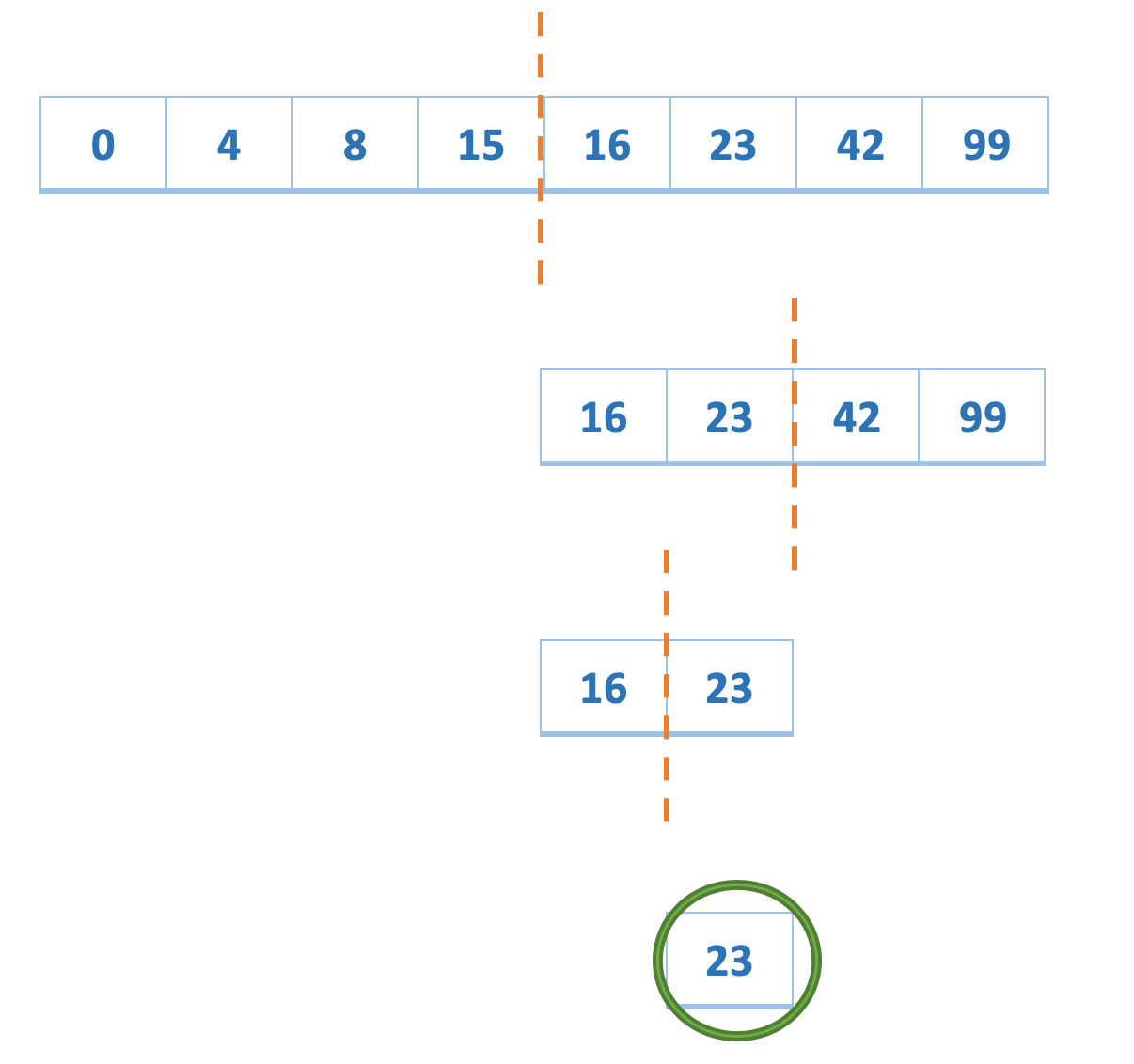Home Theory Algorithmic Paradigms – Divide and Conquer

# Algorithmic Paradigms – Divide and Conquer

1 comment

What comes to your mind when you think about divide and conquer? Do you think about wars? A strategy? A way you can split up your forces and then conquer the enemy? I am here to tell you that is exactly right.

Luckily, in computer science you don’t have to deal with weapons and artillery. There are several ways to approach a problem. Divide and conquer is one of them. In our case a problem statement is our enemy. We need to break it into parts (divide) and then solve (conquer) it. One may not realize, but you apply this algorithmic paradigm in day to day life even without realizing.

So a divide and conquer strategy to solve a problem would involve breaking down a problem into smaller, easier parts that don’t require a lot of computation. Once these small problems are solved, we are able to conquer the original problem.

##### How can I use it?

Let us take up a real life example to understand it better. You are organizing a brunch party and have 8 guests coming over. You have a loaf of bread and you want to make equal partitions for every guest.

PROBLEM: Given a loaf of bread, you need to divide it into 1/8th pieces, without using any measuring tape.Fig: Slicing a bread into 1/8th pieces.

How would you go about cutting this loaf of bread? If you are in the kitchen you would first divide the bread into half, to get 2 one-half pieces. You would again cut these pieces into 2 each to get 4 one-fourth pieces. You would repeat this process one more time with all these 4 pieces to get 8 one-eighth pieces. The approach you took would visually look something like this:Fig: Halving a bread piece at every level.

As a human being we are better able to estimate half length rather than a 1/8th length. So we took advantage of this fact and kept on slicing the bread into half until we reached 1/8th lengths. We just used a divide a conquer approach, we divided our problem into sub-parts and solved it. One could argue that we can even directly slice the bread at 1/8th length, but human minds cannot calculate that easily.

##### Okay, but what about the algorithm?

In computer science, Binary Search is a very good example of the divide and conquer paradigm. We take advantage of the fact that the array is already sorted, and keep on dividing the problem into smaller parts.

PROBLEM: Use binary search to check if the number 23 exists in the array.
arr = {0, 4, 8, 15, 16, 23, 42, 99}

• Just like the loaf of bread, we start from the middle of the array.
• All elements smaller than equal to 15 are on the left part.
• Since 23 is greater than 15, we can be pretty sure that we don’t need to search over there.
• We look into the right part.
• Again slice the array in 2 parts. All elements greater than equal to 42 are in the right part. Since 42 is greater than 23 we can ignore the right part of the array.
• Look in the left part.
• Again slice in 2 parts. Now we find our number and hence we can say that 23 exists in the array.Fig: Example of divide and conquer in a sorted array

We divided the problem into smaller parts and then conquered it using some known truth (sorted array in this case). This is how a divide and conquer paradigm can be used to solve complex problems. Some examples where we use divide and conquer are:

1 comment

#### 1 commentOctober 25, 2020 - 04:10

Thank you sir

This site uses Akismet to reduce spam. Learn how your comment data is processed.

This website uses cookies to improve your experience. We'll assume you're ok with this, but you can opt-out if you wish. Accept Read More